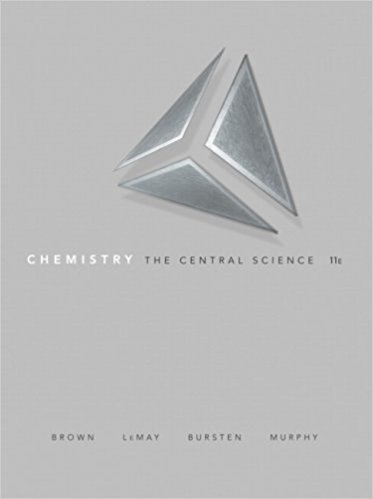×
×

# What is the expected electron configuration for (a) Ti2 +, (b) Co3+, (c) Pd2 +, (d)ISBN: 9780136006176 365

## Solution for problem 23.46 Chapter 23

Chemistry: The Central Science | 11th Edition

• Textbook Solutions
• 2901 Step-by-step solutions solved by professors and subject experts
• Get 24/7 help from StudySoup virtual teaching assistantsChemistry: The Central Science | 11th Edition

4 5 1 421 Reviews
17
0
Problem 23.46

What is the expected electron configuration for (a) Ti2 +, (b) Co3+, (c) Pd2 +, (d) Mo3+, (e) Ru3+, (f) Ni4 +?

Step-by-Step Solution:
Step 1 of 3

▯▯▯▯▯▯▯▯▯▯▯▯▯▯▯▯▯▯▯▯▯▯▯▯▯ ▯▯▯▯▯▯▯▯▯▯▯▯▯▯▯▯▯▯▯▯▯▯▯▯▯...

Step 2 of 3

Step 3 of 3

##### ISBN: 9780136006176

Chemistry: The Central Science was written by and is associated to the ISBN: 9780136006176. The answer to “What is the expected electron configuration for (a) Ti2 +, (b) Co3+, (c) Pd2 +, (d) Mo3+, (e) Ru3+, (f) Ni4 +?” is broken down into a number of easy to follow steps, and 22 words. Since the solution to 23.46 from 23 chapter was answered, more than 214 students have viewed the full step-by-step answer. This full solution covers the following key subjects: . This expansive textbook survival guide covers 25 chapters, and 2360 solutions. This textbook survival guide was created for the textbook: Chemistry: The Central Science , edition: 11. The full step-by-step solution to problem: 23.46 from chapter: 23 was answered by , our top Chemistry solution expert on 03/09/18, 07:03PM.

Unlock Textbook Solution